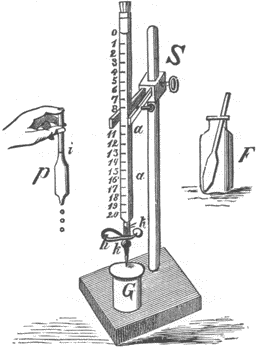Reilly, James M. The Albumen & Salted Paper Book: The history and practice of photographic printing, 1840-1895. Light Impressions Corporation. Rochester, 1980.

Titration Method for Determining Strength of Silver Nitrate SolutionsAppendix B

(Courtesy of Irving Pobboravsky)

Equipment

Centigram balance

50 ml buret

Glassware

Laboratory magnetic stirrer (optional)

Chemicals

Distilled water

Silver Nitrate, AgNO3, .1 Normal

Sodium thiocyanate, NaCNS, or Potassium thiocyanate, KCNS, .2 Normal

Ferric ammonium sulfate, saturated solution Nitric acid, 6 Molar

Process

IMPORTANT SAFETY PRECAUTION: Always wear approved laboratory eye protection and work in a well ventilated area.

STEP 1 STANDARDIZATION OF THE THIOCYANATE SOLUTION

1. Mix approximately .2 Normal sodium thiocyanate (16.2 g/liter) or potassium thiocyanate (19.4 g/liter) using distilled water. Note: Since both these chemicals are extremely hygroscopic, do not rely on the dry weight to give solutions of the correct normality. An analytical balance is not required to weigh out these amounts; a centigram balance should be sufficient.

2. Closely estimate the exact normality of the thiocyanate solution by titrating it into a silver nitrate solution of known normality:

a. Put 35 ml of 0.1 Normal silver nitrate solution into a small flask. To make a 0.1 Normal silver nitrate solution, add 4.25 g silver nitrate to enough distilled water to make a total volume of 250 ml.

b. Add 1 ml of saturated ferric ammonium sulfate solution.

c. Add 5 ml of 6 Molar nitric acid. Note: To make 6 Molar nitric acid, add 100 ml of concentrated nitric acid (15. 78N) to 163 ml of water.

d. Run in thiocyanate from a buret until a faint brownish color appears and will not disappear with vigorous stirring. Note the volume of thiocyanate at this point.

e. Calculate the normality of the thiocyanate using the following equation:

milliequivalents of silver nitrate=milliequivalents of thiocyanate

(ml1) (N1) = (ml2) (Na)

solve for N2, the normality of the thiocyanate, as follows:

 n2= (ml1)(N11) -------------------------- (ml of thiocyanate)

Example:

 n2= (35)(.1) --------- (17.5) n2= .2

STEP 2 DETERMINATION OF SILVER NITRATE CONCENTRATION

Determine the silver nitrate concentration by titrating the standardized thiocyanate solution into a sample of the silver bath as follows:

1. Put 10 ml of the silver nitrate bath into a flask. A pipet or a 10 ml cylinder graduate may be used to measure the solution.

2. Add 5 ml of 6 Molar nitric acid.

3. Add 1 ml of saturated ferric ammonium sulfate solution.

4. Run in the standardized thiocyanate solution from a buret into the silver nitrate sample until the faint brownish color will not disappear under vigorous stirring. To increase the detectability of the color, view the flask against a white background.

Note the volume of thiocyanate titrated when the color first becomes permanent, then continue adding thiocyanate drop by drop to make sure.

1. Calculate the normality of the silver bath as follows:
 normality of silver nitrate= (ml of thiocyanate) (normality of thiocyanate) ---------------------------------------------- (ml of silver nitrate in sample)

6. To obtain the concentration of silver nitrate in the bath, multiply the normality of the silver nitrate by 170 (the molecular weight of silver nitrate). The result is expressed in grams per liter.

Example:

If the normality of the silver nitrate is .65N, then:

.65(170) = 110.5 g/liter

110.5 g/liter=11.05% silver nitrate solution

7. For greater accuracy the titration procedure may be repeated and the two readings averaged together.

STEP 3 REPLENISHMENT OF THE SILVER BATH

The amount of silver nitrate required to restore the bath to proper strength will of course depend on the total volume of solution. For example, if after a titration the solution is found to be 8.5% in strength instead of 10% and possesses a total volume of 2 liters, the first step is to calculate how many grams per liter are lacking. In this case instead of 100 g/liter, there are only 85 g/liter, so 15 g/liter are missing. Multiply the number of missing grams/liter times the total number of liters of solution to find the amount of silver nitrate needed to replenish the solution:

15 g (2 liters) = 30 g needed for replenishmentFig. 47. Titration apparatus, from an 1887 manual. P is a pipet for measuring the correct volume of silver solution, S is the buret and F is the vessel for conducting the titration.

Helpful Hints for the Titration Procedure

1. Because thiocyanate solutions keep well, it is convenient to make up a large quantity of standardized solution. The ferric ammonium sulfate and nitric acid stock solutions will also keep well. Nitric acid should be stored in a ground-stoppered glass bottle.

2. Do not pour the thiocyanate solution that remains in the buret after a titration back into the container of standardized stock solution--throw it out.

3. Instead of calculating the normality each time a titration is performed, it is helpful to make a chart for each batch of standardized thiocyanate solution. The chart can be made by calculating a few points and drawing a line through the points. Label one axis of the graph in ml of standardized thiocyanate solution and the other in g/liter silver nitrate.

4. For increased economy a 5 ml sample of silver nitrate bath can be used without sacrificing accuracy, providing the sample is carefully measured out.